BGonline.org Forums

Not a favorite, leading match: cube action? - RO

Posted By: Daniel Murphy
Date: Saturday, 5 October 2013, at 11:28 p.m.

In Response To: Not a favorite, leading match: cube action? (Daniel Murphy)

Blue on roll.

White2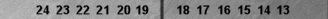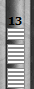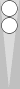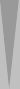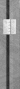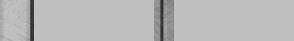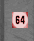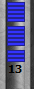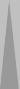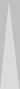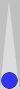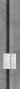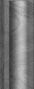Blue7
Position ID: AwAAoAAAAAAAAA Match ID: cAngACAAGAAE

Blue is not a favorite to bear off, winning exactly 17/36 = 47.22%. An obvious nondouble for money. But at all pre-Crawford 5-point match scores, Blue should double if he is leading in the match, and should not double if he is tied or trailing.

 1-away 2-away 3-away 4-away 5-away 1-away X X X X X 2-away DT ND DT DT DT 3-away DT ND ND DT DT 4-away DT ND ND ND DT 5-away DT ND ND ND ND

Score 2a5a: Double, Lose -> 2a3a for 60%, No Double, Lose -> 2a4a for 67%, Risk = 7%, Double, Win -> 0a5a for 100%, No Double, Win -> 1a5a for 84%, Gain = 16%, R/(R+G) = 30.43%

Score 2a4a: Double, Lose -> 2a2a for 50%, No Double, Lose -> 2a3a for 60%, Risk = 10%, Double, Win -> 0a4a for 100%, No Double, Win -> 1a4a for 81%, Gain = 19%, R/(R+G) = 34.48%

Score 3a5a: Double, Lose -> 3a3a for 50%, No Double, Lose -> 3a4a for 57%, Risk = 7%, Double, Win -> 1a5a for 84%, No Double, Win -> 2a5a for 74%, Gain = 10, R/(R+G) = 41.11%

Score 3a4a: Double, Lose -> 3a2a for 40%, No Double, Lose -> 3a3a for 50%, Risk = 10%, Double, Win -> 1a4a for 81%, No Double, Win -> 2a4a for 67%, Gain = 14%, R/(R+G) = 41.66%

Score 2a3a: Double, Lose -> 2a1a for 32%, No Double, Lose -> 2a2a for 50%, Risk = 18%, Double, Win -> 0a3a for 100%, No Double, Win -> 1a3a for 75%, Gain = 25%, R/(R+G) = 41.86%

Score 4a5a: Double, Lose -> 4a3a for 43%, No Double, Lose -> 4a4a for 50%, Risk = 7%, Double, Win -> 2a5a for 74%, No Double, Win -> 3a5a for 65%, Gain = 9%, R/(R+G) = 43.75%

In words, Blue's GWC is above his last-roll doubling point at all the scores above, so Blue should double now.

Alternatively,

Score No Double Equity Double/Take Equity
2a3a (17/36 * 75%) + (19/36 * 50%) = 61.80% (17/36 * 100% + (19/36 * 32%) = 64.11%
2a4a (17/36 * 81%) + (19/36 * 40%) = 59.36% (17/36 * 100% + (19/36 * 50%) = 73.61%
2a5a (17/36 * 84%) + (19/36 * 67%) = 75.03% (17/36 * 100% + (19/36 * 60%) = 78.88%
3a4a (17/36 * 67%) + (19/36 * 50%) = 58.03% (17/36 * 81% + (19/36 * 40%) = 59.36%
3a5a (17/36 * 74%) + (19/36 * 57%) = 65.03% (17/36 * 84% + (19/36 * 50%) = 66.05%
4a5a (17/36 * 65%) + (19/36 * 50%) = 57.08% (17/36 * 74% + (19/36 * 43%) = 57.63%

Since Blue's D/T equity is higher than his ND equity, Blue should double.

Post Response

Subject:
Message: